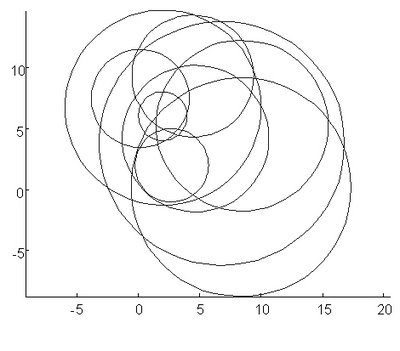## 10/25/2006

### 6.4次函數

`function drawcircles(r) % 主函數% Draw circles with radii of r.global MAXR;n=length(r);MAXR=max(r);hold on;for i=1:n,   [X,Y]=circ(r(i));   plot(X,Y);endhold off;axis equal;endfunction [x,y]=circ(rr)%次函數% Calculate the points of a circle.   [xx,yy]=randxy;   theta=linspace(0,2*pi,60);   x=xx+rr*cos(theta);   y=yy+rr*sin(theta);endfunction [xx,yy]=randxy%次函數global MAXR% locate the position of the center.xx=rand*MAXR;yy=rand*MAXR;end`

drawcircles(2:10)